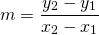# Using Slope and y intercept (Skill #4)

Use the formulato find the slope of each line. Use the vertical intercept to complete the equation in the formWorked Example: example slope y intercept